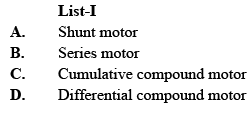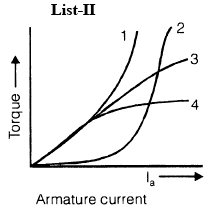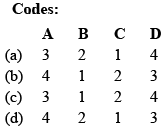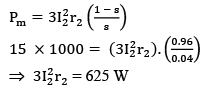Courses

# Electrical Machines - MCQ Test 1

## 10 Questions MCQ Test GATE Electrical Engineering (EE) 2022 Mock Test Series | Electrical Machines - MCQ Test 1

Description
This mock test of Electrical Machines - MCQ Test 1 for GATE helps you for every GATE entrance exam. This contains 10 Multiple Choice Questions for GATE Electrical Machines - MCQ Test 1 (mcq) to study with solutions a complete question bank. The solved questions answers in this Electrical Machines - MCQ Test 1 quiz give you a good mix of easy questions and tough questions. GATE students definitely take this Electrical Machines - MCQ Test 1 exercise for a better result in the exam. You can find other Electrical Machines - MCQ Test 1 extra questions, long questions & short questions for GATE on EduRev as well by searching above.
QUESTION: 1

Solution:
QUESTION: 2

### Consider the following statements: The use of interpoles in dc machines is to counteract the 1. reactance voltage 2. demagnetizing effect of armature mmf in the commutating zone 3. cross-magnetizing effect of armature mmf in the commutating zone Which of these statement(s) is/are correct?

Solution:

In practice, the interpoles of appropriate polarity are strengthened so that in interpolar zone, the armature cross flux is neutralized and in addition some flux is produced there. This additional flux in the interpolar zone induces rotational emf in the commutated coil in such a direction as to oppose the reactance voltage. So, the resultant emf in the commutated coil would be zero and therefore zero current in that coil would amount to sparkless commutation. This is the reason why interpoles are designed to provide more mmf than the armature mmf in the commutating zone. In practice, the interpole mmf may be 1.2 to 1.3 times the armature mmf per pole.

QUESTION: 3

### In an armature, if m is the number of slots per pole per phase and γ is the slot pitch angle, then the breadth or the distribution factor for the armature winding is

Solution:
QUESTION: 4

The current drawn by a 220V dc motor of armature resistance 0.5 Ω and back emf 200V is

Solution:

Ea = V – Ia Ra
Ea = 200 = 220 – Ia × 0.5 ⇒ Ia = 40 A

QUESTION: 5

A dc shunt generator when driven without connecting field winding shows an open circuit terminal voltage of 12V. When field winding is connected and excited, the terminal voltage drops to zero because

Solution:

If shunt field resistance is more than the critical field resistance, there will be no voltage build up beyond residual voltage but voltage would not be zero.

QUESTION: 6

Match List-I (dc motors) with List-II (Characteristics) labeled 1, 2, 3 and 4) and select the correct answer:Solution:
QUESTION: 7

An induction motor when started on load does not accelerate up to full speed but runs at 1/7th of the rated speed. The motor is said to be

Solution:

Refer, harmonic asynchronous (induction) torques. Running stably at low speed (around 1/7th normal motor speed) is known as Crawling.

QUESTION: 8

The rotor power output of 3-phase induction motor is 15 kW. The rotor copper losses at a slip of 4% will be

Solution:QUESTION: 9

Skewing of the rotor in a three-phase squirrel-cage induction motor reduces

Solution:

If rotor slots are skewed by 2 harmonic polepitches, then effect of slot harmonics (Parasitic torques) can be eliminated.
Since the skewed rotor slot is not parallel to stator slot in an induction motor, there is more leakage reactance. As a result, induction motor has lower starting and maximum torques.
With skewed rotor bars, the revolving flux, in effect, encounters an air gap of uniform reluctance and this results in a uniform torque and quieter operation.

QUESTION: 10

In a shaded-pole induction motor, the rotor runs from the

Solution: